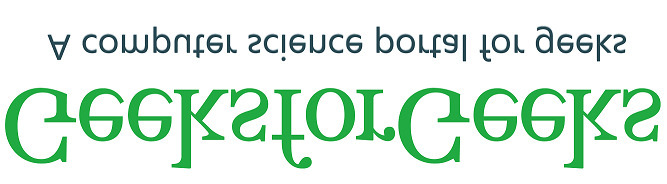Skip to content
Related Articles
Wand flip() function – Python
• Last Updated : 19 May, 2021

The flip() function is an inbuilt function in the Python Wand ImageMagick library which is used to create a vertical mirror image by reflecting the pixels around the central x-axis.

Syntax:

`flip()`

Parameters: This function does not accept any parameters.
Return Value: This function returns the Wand ImageMagick object.

Original Image:Example 1:

## Python3

 `# Import library from Image``from` `wand.image ``import` `Image` `# Import the image``with Image(filename ``=``'../geeksforgeeks.png'``) as image:``    ``# Clone the image in order to process``    ``with image.clone() as flip:``        ``# Invoke flip function``        ``flip.flip()``        ``# Save the image``        ``flip.save(filename ``=``'flip1.jpg'``)`

Output:Example 2:

## Python3

 `# Import libraries from the wand ``from` `wand.image ``import` `Image``from` `wand.drawing ``import` `Drawing``from` `wand.color ``import` `Color` `with Drawing() as draw:``    ``# Set Stroke color the circle to black``    ``draw.stroke_color ``=` `Color(``'black'``)``    ``# Set Width of the circle to 2``    ``draw.stroke_width ``=` `1``    ``# Set the fill color to 'White (# FFFFFF)'``    ``draw.fill_color ``=` `Color(``'white'``)` `    ``# Invoke Circle function with center at 50, 50 and radius 25``    ``draw.circle((``200``, ``200``), ``# Center point``                ``(``100``, ``100``)) ``# Perimeter point``    ``# Set the font style``    ``draw.font ``=` `'../Helvetica.ttf'``    ``# Set the font size``    ``draw.font_size ``=` `30``    ` `    ``with Image(width ``=` `400``, height ``=` `400``, background ``=` `Color(``'# 45ff33'``)) as pic:``        ``# Set the text and its location``        ``draw.text(``int``(pic.width ``/` `3``), ``int``(pic.height ``/` `2``), ``'GeeksForGeeks !'``)``        ``# Draw the picture``        ``draw(pic)``        ``# Invoke flip() function``        ``pic.flip()``        ``# Save the image``        ``pic.save(filename ``=``'flip2.jpg'``)`

Output:Attention geek! Strengthen your foundations with the Python Programming Foundation Course and learn the basics.

To begin with, your interview preparations Enhance your Data Structures concepts with the Python DS Course. And to begin with your Machine Learning Journey, join the Machine Learning – Basic Level Course

My Personal Notes arrow_drop_up